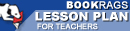Lesson Plans

# The Logic of Scientific Discovery Test | Mid-Book Test - Easy

This set of Lesson Plans consists of approximately 102 pages of tests, essay questions, lessons, and other teaching materials.
 View a FREE sampleName: _________________________ Period: ___________________

This test consists of 15 multiple choice questions and 5 short answer questions.

## Multiple Choice Questions

1. What can observation do for science?
(a) Establish the truth of a statement.
(b) Provide information on facts.
(c) Justify statements.
(d) Assume falsification.

2. What comprises empirical science?
(a) Systems of theories.
(b) Guides to explanations.
(c) Natural laws.
(d) Universal assumptions.

3. What does Popper say methodological rules are?
(a) Keys to understanding.
(b) Testable.
(c) Conventions.
(d) Irrelevant.

4. Statements that rule things out rather than include more things are considered what type of statements?
(a) Falsifiable.
(b) Universal.
(c) Tautological.
(d) Singular.

5. What types of statements are scientific theories?
(a) Singular.
(b) Universal.
(c) Logical.
(d) Proven.

6. How do conventionalists view Poppers beliefs on the underpinnings of new discoveries?
(a) Unwarranted.
(b) A collapse of science.
(c) Logical.
(d) Good judgment.

7. Natural laws are in a form to negate what type of statements?
(a) Proven.
(b) Existential.
(c) Singular.
(d) Universal.

8. For what purpose are methodological rules developed?
(a) Question demarcation.
(b) Protect demarcation.
(c) Wholly prove demarcation.
(d) Falsity demarcation.

9. What is the theory of theories?
(a) System of theories.
(b) Existential statements.
(c) The logic of science.
(d) Causality.

10. According to conventionalists, what will yields more complete results of science?
(a) Logical constructions of nature.
(b) Greater mastery.
(c) Inferences.
(d) Experimentation.

11. What happens when theories are refined?
(a) They become obsolete.
(b) They become proven fact.
(c) Science prevails.
(d) They become better explanatory tools.

12. What deductive tests compare conclusions?
(a) Comparing theories.
(b) Internal consistency.
(c) Empirical applications.
(d) Investigations.

13. What challenges Poppers use of falsification as criteria in deciding is a theoretical system is empirical?
(a) Conventionalism.
(b) Construction of Nature.
(c) Competence.
(d) Theoretical experiments.

14. What depends on both universal and singular statements?
(a) Correlations.
(b) Causality.
(c) Logical factors.
(d) Abstract thought.

15. What is the reason Popper rejects psychologism?
(a) Perceptions do not allow for justifications.
(b) Perceptions are inter-subjective.
(c) Perceptions are inter-objective.
(d) Perceptions allow for justifications.

1. How does logic serve in determining if a statement is testable?

2. What refers to a term that does not need a definition but is learned through experience?

3. What confuses the distinction between universal and individual names?

4. What is diametrically opposed to the critical attitude required of a scientist?

5. What does Popper consider to contribute to the cosmology of empirical science?

 This section contains 362 words (approx. 2 pages at 300 words per page) View a FREE sample